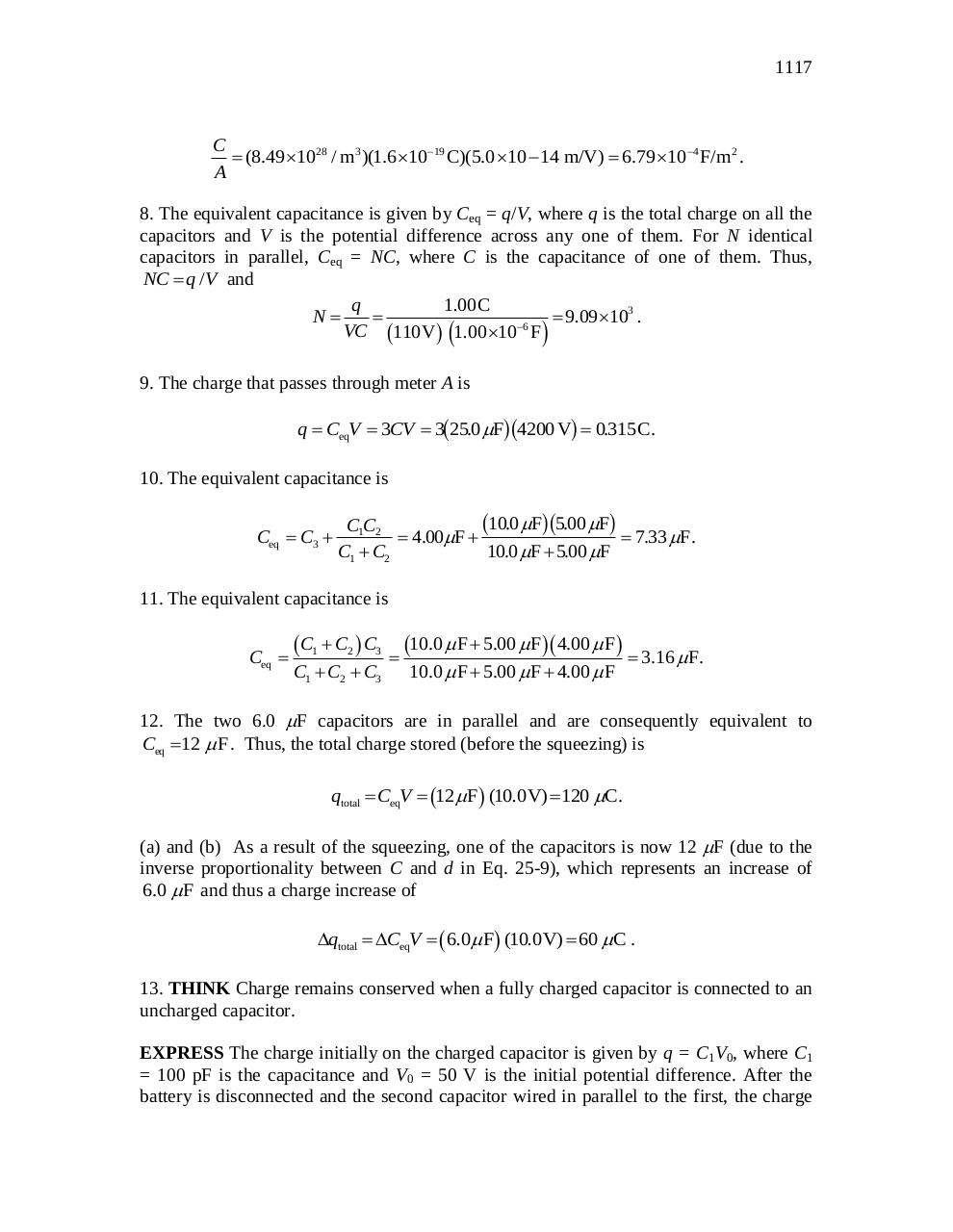# chapter 25.pdfPage 1 2 34532

#### Text preview

1117

C
 (8.49 1028 / m3 )(1.6 1019 C)(5.0 10  14 m/V)  6.79 104 F/m2 .
A

8. The equivalent capacitance is given by Ceq = q/V, where q is the total charge on all the
capacitors and V is the potential difference across any one of them. For N identical
capacitors in parallel, Ceq = NC, where C is the capacitance of one of them. Thus,
NC  q / V and
q
1.00C
N

 9.09 103 .
6
VC 110V  1.00 10 F 
9. The charge that passes through meter A is

b

gb

g

q  CeqV  3CV  3 25.0 F 4200 V  0.315 C.
10. The equivalent capacitance is
Ceq  C3 

b

gb

g

10.0 F 5.00 F
C1C2
 4.00F 
 7.33 F.
C1  C2
10.0 F  5.00 F

11. The equivalent capacitance is
Ceq 

 C1  C2  C3  10.0  F  5.00  F 4.00  F  3.16  F.
C1  C2  C3

10.0  F  5.00  F  4.00  F

12. The two 6.0 F capacitors are in parallel and are consequently equivalent to
Ceq  12  F . Thus, the total charge stored (before the squeezing) is

qtotal  CeqV  12  F (10.0V)  120 C.
(a) and (b) As a result of the squeezing, one of the capacitors is now 12 F (due to the
inverse proportionality between C and d in Eq. 25-9), which represents an increase of
6.0  F and thus a charge increase of

qtotal  CeqV   6.0  F (10.0V)  60 C .
13. THINK Charge remains conserved when a fully charged capacitor is connected to an
uncharged capacitor.
EXPRESS The charge initially on the charged capacitor is given by q = C1V0, where C1
= 100 pF is the capacitance and V0 = 50 V is the initial potential difference. After the
battery is disconnected and the second capacitor wired in parallel to the first, the charge